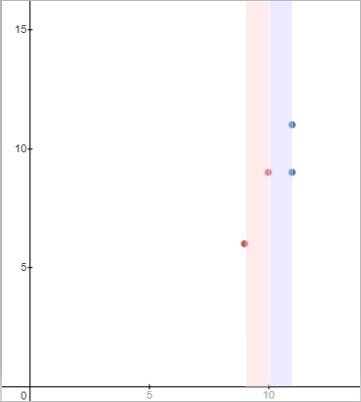# Program to find out the vertical area between two points where no point lies and is the widest in Python

Suppose, we are given n number of points as (x, y). A vertical area is an area that is extended infinitely along the y-axis. We have to find out the vertical area between two points such that no other point is inside the area and is the widest.

So, if the input is like pts = [[10,9],[11,11],[9,6],[11,9]], then the output will be 1.The areas in red and blue are optimal and there are no points inside them.

To solve this, we will follow these steps −

• sort the list pts

• for i in range 1 to size of pts, do

• return the maximum value of (pts[i, 0] - pts[i - 1, 0])

## Example

Let us see the following implementation to get better understanding

def solve(pts):
pts.sort()
return max(pts[i] - pts[i - 1] for i in range(1, len(pts)))
print(solve([[10,9],[11,11],[9,6],[11,9]]))

## Input

[[10,9],[11,11],[9,6],[11,9]]


## Output

1